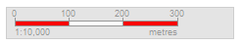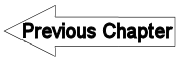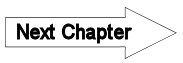# A2.6 Scale - LUPMISManual

Go to content

Annexes 1-10 > A2. Background to cartography/raster images

A2.6 Scale

Level of expertise required for this Chapter: Beginner; general GIS training

The scale of a map is usually defined as the ratio of a single unit of distance on the map to the corresponding distance on the ground.

For example, a scale of 1:2,500 means: 1 cm on the map is equivalent to 2500 cm (= 25 m) on the ground.

True ground distances are calculated by measuring the distance on the map (in any unit) and multiplying times the inverse of the scale fraction. True ground areas are calculated by measuring the area on the map (in any unit) and then multiplying time the inverse of the scale fraction squared.

Large scale’ and ‘small scale’:

In LUPMP, the scale of 1:2,500 is considered large whilst a scale of 1:200,000 is a small scale. There is no hard and fixed dividing line between small and large scales.

Examples:

 National map 1:1,000,000 Very small scale District map,Framework plan 1:100,000 Small scale Township map, Structure Plan 1:25,000 Medium scale Sector map,Local plan 1:2500 Large scale Parcel map,Site plan 1:1000 Very large scale

- - - - -

The scale number is the inverse of the scale fraction. It is the denominator of the scale fraction. For example, a scale of 1:2500,which implies 1 / 2500, has a 2500 as the scale number.

- - - - -

The screen scale is the scale, at which the feature is displayed at the Map Maker screen. It is normally shown on the bottom left of the Map Maker screen: